### MEMO 2018 ekipno problem 4

Kvaliteta:
Avg: 0,0
Težina:
Avg: 6,0

Let$n$ be a positive integer and let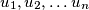$u_1, u_2, \ldots u_n$ be positive integers not larger than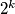$2^k$ for some integer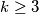$k \geq 3$. A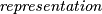$\emph{representation}$ of a non-negative integer$t$ is a sequence of non-negative integers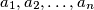$a_1, a_2, \ldots, a_n$ such that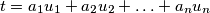Prove that if a non-negative integer$t$ has a representation, then it also has a representation where less than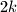$2k$ of the numbers$a_1, a_2, \ldots, a_n$ are non-zero.

Izvor: Srednjoeuropska matematička olimpijada 2018, ekipno natjecanje, problem 4